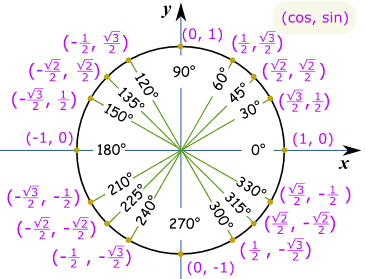# How do you find the value of cos 300?

Aug 4, 2016

$= 0.5$

#### Explanation:

$\cos 300$
$= 0.5$

Aug 4, 2016

$\cos \left({300}^{\circ}\right) = \frac{1}{2}$

#### Explanation:

Imagine the unit circle:We know that ${300}^{\circ}$ is in the fourth quadrant, where cosine is positive.

${300}^{\circ}$ has a reference angle of ${60}^{\circ}$, since it is ${60}^{\circ}$ away from the $x$-axis.

Since $\cos \left({60}^{\circ}\right) = \frac{1}{2}$, we know that $\cos \left({300}^{\circ}\right) = \frac{1}{2}$ as well since $\cos \left(\theta\right) > 0$ in the fourth quadrant.